# Reasoning: Identifying Opportunities (Article)

##### Age 5 to 11

Published 2014 Revised 2022

This article, written by Jennie, Liz and Bernard, forms part of our Reasoning Feature, and complements the article Reasoning: The Journey from Novice to Expert.

#### Introduction

Reasoning is fundamental to knowing and doing mathematics. We wonder how you would define the term? Some would call it systematic thinking. Reasoning enables children to make use of all their other mathematical skills and so reasoning could be thought of as the 'glue' which helps mathematics makes sense.

The second aim of the mathematics national curriculum in England (DfE, 2013) is that all pupils will:

reason mathematically by following a line of enquiry, conjecturing relationships and generalisations, and developing an argument, justification or proof using mathematical language.

In order to explore this aim, three questions need to be answered:

1. When is reasoning necessary?
2. What do we do when we reason?
3. How do we support children to develop their reasoning skills?

In this article, we discuss the first of these questions in depth and this leads to consideration of the second. We outline eight contexts in which we believe reasoning is required, using tasks from the NRICH site to illustrate each. This helps to highlight how reasoning is the 'glue' that helps mathematics make sense. It also helps us to begin to think about how we could support children to develop their reasoning skills (question 3) once we have ascertained where these are needed and what they might look like. The article Reasoning:  The Journey from Novice to Expert explores question 3 in depth.

#### When is reasoning needed?

1.  When first encountering a new challenge
When faced with a mathematical challenge, reasoning helps us to make use of relevant prior knowledge such as how to tackle this 'type' of problem or a particular calculation method that could prove useful. The reasoning involved is complex and unique to the individual, as each of us has a different mix of past mathematical experiences.

2.  When logical thinking is required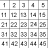If you are not familiar with it, have a go at the Hundred Square challenge. In this activity, a hundred square has been printed on both sides of a piece of paper with one square directly behind the other. The idea is to find out what number is on the back of various chosen numbers.

This activity demands logical thinking in order to convince ourselves (and others) which number is behind the given number. A solution might involve a chain of statements, which follow on from one another. For example, we received the following from Luke who describes his logic when finding out what is on the back of 23:

If the number is 23 then it is placed two places away from the end of the line.
Next you go two places back from the other end of the same line to get the correct answer of 28. Like 19 would be 12 on the other side of the sheet.

3.  When a range of starting points is possible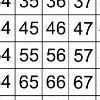In That Number Square, a hundred square has fallen down. The blank square remains on the wall with a heap of numbers on the floor. The challenge is to find a quick way of putting back all the numbers.

In this case, there is not one single, obvious starting point and so reasoning is required before we even begin the task. Drawing on prior knowledge, perhaps we would select some 'benchmarks', for example we might put in all the numbers ending in zero first, or choose to position one number from each row first, or place 0 or 1 or 99 or 100? Alternatively, we might simply pick numbers up at random and slot them in to save time hunting for a particular one. Once we have used reasoning to select a starting point and have therefore placed at least one number, our reasoning might make use of the number/s already in position, drawing on our knowledge of patterns and relationships in the hundred square.

4.  When there are different strategies to solve a problem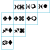Sticking with the hundred square theme, Coded Hundred Square is an example of a task which can be solved in a number of different ways. This time, the hundred square is presented as a coded jigsaw and the idea is to complete it.

One solution we received, from Anna, describes her method:

I knew that the square went from 1 to 100. That meant there was a number that had 3 digits (100). I worked out that all of the numbers on the first row only had one digit so I started from the first row and worked my way on.

By contrast, Rebecca used a completely different strategy:

The way I worked it out was easy I just forgot about the numbers and the code and I just fitted the shapes in the way that they would go, and at the end I worked out the code and the numbers were right.

Making the decision as to how to tackle the activity necessarily involves reasoning.

5.  When there is missing information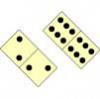In Amy's Dominoes, Amy thinks that some dominoes are an incomplete set. She has 24 dominoes in her box and there are 125 spots on them altogether. We are lacking information needed to solve the task - how many dominoes are there in a complete set? How many spots are there altogether in a complete set?

Reasoning is required on three levels here. Firstly, we have to use reasoning to recognise that information is missing. Secondly, we use reasoning to figure out what it is that we need to know.  Thirdly, we use reasoning to draw on our existing knowledge and to work out that information.

6.  When selecting a problem-solving skill
In order to solve a problem, we need to draw on one or more problem-solving skills, such as:
• Working systematically
• Trial and improvement
• Logical reasoning
• Spotting patterns
• Visualising
• Working backwards
• Conjecturing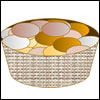When presented with a problem, we need to decide which problem-solving skill to try first. This might be a conscious decision for some, whereas others may simply 'plump' for the first skill that springs to mind.

When presented with the problem Eggs in Baskets, we may choose to adopt a trial and improvement approach. For example, Erika sent us this solution:

If there was 1 egg in the brown basket, no eggs in the red basket and 3 eggs in the pink basket, that would only make 4 eggs.
If there were 2 eggs in the brown basket, 1 egg in the red basket and 4 eggs in the pink basket, this only makes 7 eggs.
So finally, if there were 3 eggs in the brown basket, 2 eggs in the red basket and 5 eggs in the pink basket, there would be 10 eggs altogether.

However, Jas also sent his solution to us, but he chose to use an algebraic approach:

Total eggs = 10, so
n + (n+1) + (n+3) = 10
3n + 4 = 10
3n = 6
n = 2

The reasoning involved in selecting a problem-solving skill (whether conscious or unconscious) might draw on past experience ("I've seen something like this before and I remember I did this ..."). If the situation feels very unfamiliar then trial and improvement might be chosen as a 'way in'.

7.  When evaluating a solution in context
Having come to a solution, it is valuable to reflect on whether the answer is 'sensible' in the context of the problem.  Have a go at the two questions below:

56 children are going to a football match.
A minibus can take 12 children.
How many minibuses do I need?

Eggs are sold in boxes of 6.
I have 37 eggs.
How many boxes can I sell?

In each case, a division calculation is necessary but we will need to make sense of the answer we obtain. 4 remainder 8 buses? 6.17 boxes? Reasoning tells us that these are meaningless answers. Further reasoning helps us take into account the contexts and therefore prompts us to interpret our solutions and tweak them.

8.  When there is more than one solution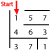In Maze 100, the challenge is to find a route through the grid so that the cumulative total of the numbers we have passed through is 100. Having found one solution, we might ask ourselves whether there are any others. Do we use reasoning to tweak the solution we have already found in search of another, or do we start from scratch?

If the challenge is to find all possible solutions, then the reasoning might involve having a system which ensures none are left out.

What do we do when we reason?

Having discussed some occasions when reasoning is necessary, we hope that your understanding of what reasoning 'looks like' has deepened.  It is difficult to articulate what exactly reasoning is but, drawing on the above, here are some suggestions about what we do when we reason:
• Evaluate situations
• Select problem-solving strategies
• Draw logical conclusions
• Develop solutions
• Describe solutions
• Reflect on solutions
This list is not exhaustive but it might help you talk about reasoning in the context of the classroom.

In summary

In this article, we have discussed when reasoning may be needed in the problem-solving process both to highlight how reasoning is the 'glue' that helps mathematics make sense and also to help us to refine our understanding of what we mean by the term reasoning. How has your definition of reasoning changed as you have read this article? Once we have a clear understanding of what reasoning 'looks like' then we can begin to think about how we can support children to develop their reasoning skills. We explore this further the article Reasoning: The Journey from Novice to Expert.